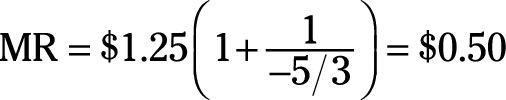##### Microeconomics For Dummies - UK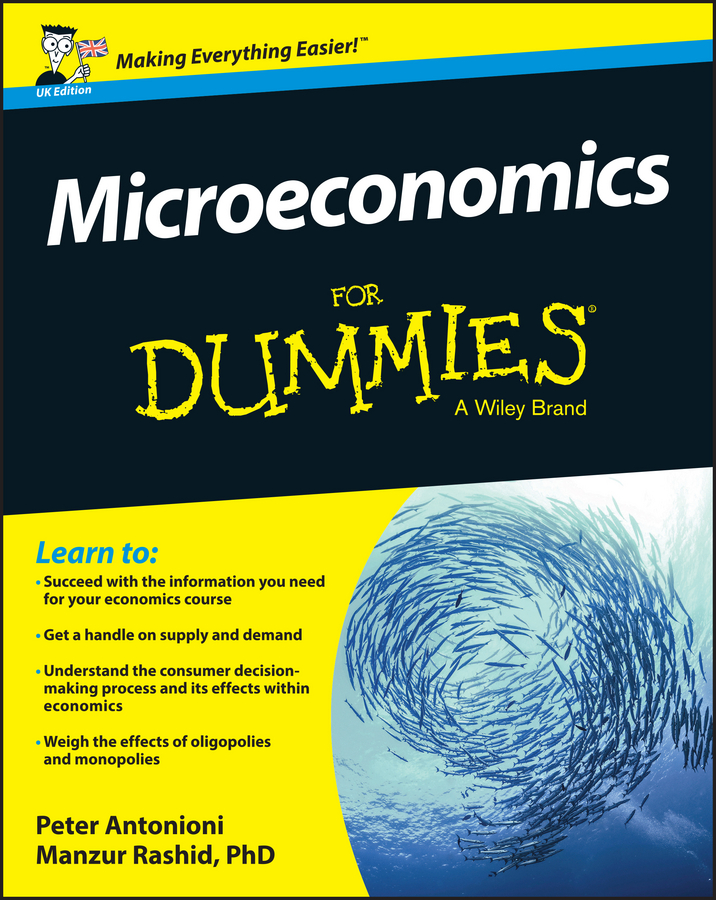Total revenue equals the good’s price multiplied by the quantity sold. Because the price elasticity of demand shows the relationship between price and quantity sold, the elasticity number captures all the information you need to anticipate changes in total revenue.

If demand is inelastic (the price elasticity of demand is between 0 and –1), the quantity sold does not change very much when price changes. As a result, a higher price causes a very small decrease in the quantity sold and total revenue increases. (The higher price you receive for the goods you sell more than offsets the slightly smaller number you sell.)

On the other hand, charging a lower price does not cause much of an increase in quantity demanded; total revenue decreases.

As these situations illustrate, when demand is inelastic, price and total revenue change in the same direction; they both increase or decrease together.

For an elastic demand (the price elasticity of demand is bigger than –1), the opposite situation occurs; price and total revenue move in opposite directions. (The higher price you receive isn’t enough to offset the decrease in the amount of goods you sell.) If you decrease the good’s price, a large increase occurs in quantity demanded, and total revenue increases.

Thus, when demand is elastic, price and total revenue change in opposite directions.

Say that your vending machine company initially charges a \$1.50 per bottle and sells 2,000 bottles per week. Decreasing the price to \$1 increases sales to 4,000 bottles. The price elasticity of demand equals –1.67; demand is elastic.

The price decrease increases total revenue from \$3,000 to \$4,000 because the \$0.50 decrease in price is more than offset by selling 2,000 more bottles. Thus, price and total revenue move in opposite directions given the elastic demand.

Another useful concept is marginal revenue, the change in total revenue that occurs when one additional unit of a good is sold. The formula describing the relationship between marginal revenue, MR, and the price elasticity of demand, η, is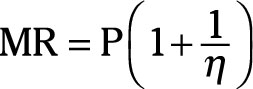Use this formula with the point price elasticity of demand.

If your good is currently selling at price P, and you know the point price elasticity of demand η, you can quickly determine how much your revenue changes if you lower price to sell one additional unit of the good.

Assume that your company charges a \$1.25 per bottle of soft drink and the point price elasticity of demand is –5/3. To determine marginal revenue (the amount of revenue you add by selling one more bottle):

1. Insert \$1.25 for P and -5/3 for ç.

2. Calculate the value in the parentheses.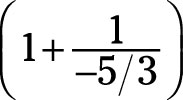equals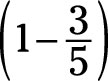or 2/5.

3. Multiply \$1.25 by 2/5.

The marginal revenue equals \$0.50.

So the marginal revenue received when an additional bottle is sold is